## Percent Solutions

### Learning Objectives

• Define concentration.
• Perform calculations involving mass percent and volume percent solutions.

### How many is too much?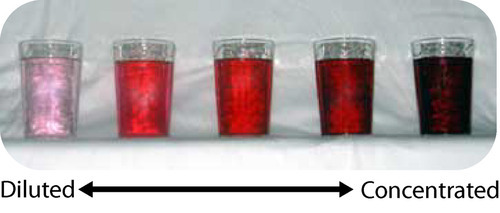Image by FirstPrinciples/Wikimedia Commons.

There are cultures that have no numbers above three. Anything greater than that is simply referred to as “much” or “many”. We recognize how limited this form of calculation is, but we do some of the same thing. There are several ways to express the amount of solute in a solution in a quantitative manner. The concentration of a solution is a measure of the amount of solute that has been dissolved in a given amount of solvent or solution. A concentrated solution is one that has a relatively large amount of dissolved solute. A dilute solution is one that has a relatively small amount of dissolved solute. However, those terms are vague and we need to be able to express concentration with numbers.

## Percent Solutions

One way to describe the concentration of a solution is by the percent of a solute in the solvent. The percent can further be determined in one of two ways: (1) the ratio of the mass of the solute divided by the mass of the solution or (2) the ratio of the volume of the solute divided by the volume of the solution.

## Mass Percent

When the solute in a solution is a solid, a convenient way to express the concentration is a mass percent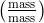, which is the grams of solute per 100 g of solution.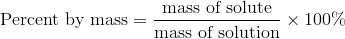Suppose that a solution was prepared by dissolving 25.0 g of sugar into 100 g of water. The percent by mass would be calculated by: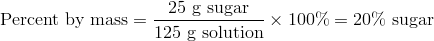Sometimes you may want to make up a particular mass of solution of a given percent by mass and need to calculate what mass of the solvent to use. For example, you need to make 3000 g of a 5% solution of sodium chloride. You can rearrange and solve for the mass of solute.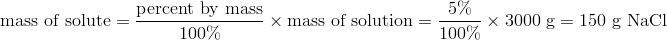You would need to weigh out 150 g of NaCl and add it to 2850 g of water. Notice that it was necessary to subtract the mass of the NaCl (150 g) from the mass of solution (3000 g) to calculate the mass of the water that would need to be added.

## Volume Percent

The percentage of solute in a solution can more easily be determined by volume when the solute and solvent are both liquids. The volume of the solute divided by the volume of solution expressed as a percent yields the percent by volume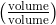of the solution. If a solution is made by adding 40 mL of ethanol to 20 mL of water, the percent by volume is: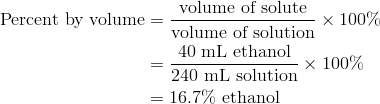Frequently, ingredient labels on food products and medicines have amounts listed as percentages (see figure below).

### Summary

• Techniques for calculation percent mass and percent volume solution concentrations are described.

### Practice

Read pages 1-3 of the material on the link below and do the problems associated with that section. Work the problems before checking the answers.

http://go.hrw.com/resources/go_sc/mc/HUGPS163.PDF

### Review

1. Why are the terms “concentrated” and “dilute” not very helpful?
2. When would you use volume percent calculations?
3. Why do you subtract the mass of the solute from the total mass desired when making mass percent calculations?

## Glossary

• concentrated: A solution that has a relatively large amount of dissolved solute.
• concentration: A measure of the amount of solute that has been dissolved in a given amount of solvent or solution.
• dilute: A solution that has a relatively small amount of dissolved solute.